The SIMILARITY Procedure

The working input sequence can be scaled to the working target sequence. Sequence scaling is applied after normalization. Let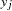be the working target sequence with mean,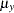, and standard deviation,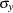. Let
be the working input sequence and let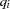be the scaled sequence. The scaling is defined as follows:

• Standard is the standard normalization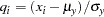• Absolute is the absolute scaling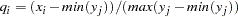• User-Defined is a user-defined scaling

Note: This procedure is experimental.Previous Page | Next Page | Top of Page